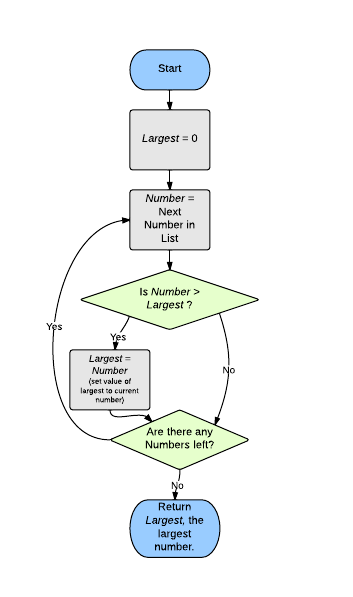# 3 ways to show an Algorithm

To find the largest number, you could have given instructions like these:

1. Take a paper and pencil and put a 0 on it. Name the number stored on the paper largest.
2. Compare each number in the submitted numbers with largest. If any number is greater than largest, erase largest and replace it with number.
3. When you finish going through all the numbers, the value of largest will be the greatest number.

Even for a simple task like finding a large number, you need to give precise instructions. These instructions could also be demonstrated in a flowchart:The above instructions can also be written in psuedo-code, which is similar to real code:

(input: a list of numbers)

``````largest = 0
for each number in numbers:
if number > largest:
largest = number  //(this assigns the value of number to largest)
return largest
``````

These are 3 different ways to show the same algorithm for a simple task. They should give you an idea of what it means to program a computer - to write step-by-step exact instructions for the computer to follow to accomplish a task.

### Challenge

What will the following algorithm return when given the following list of numbers: {1,2,3,5}

``````ttl = 0  //(assigns value of 0 to 'ttl')
for each number in numbers:
ttl = (ttl + number)   //assigns new value to 'ttl'
return ttl
``````